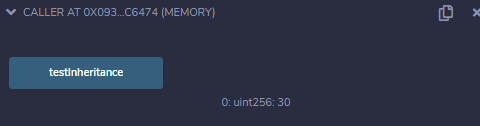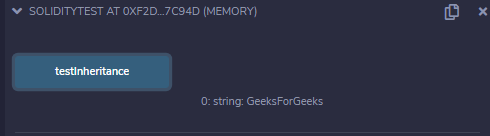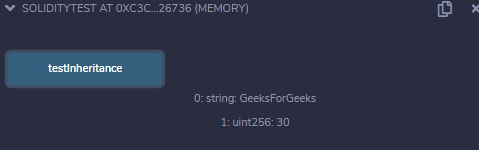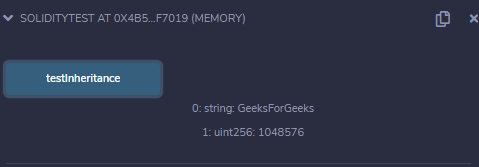Related Articles

# Solidity – Inheritance

Inheritance is one of the most important features of the object-oriented programming language. It is a way of extending the functionality of a program, used to separate the code, reduces the dependency, and increases the re-usability of the existing code. Solidity supports inheritance between smart contracts, where multiple contracts can be inherited into a single contract. The contract from which other contracts inherit features is known as a base contract, while the contract which inherits the features is called a derived contract. Simply, they are referred to as parent-child contracts. The scope of inheritance in Solidity is limited to public and internal modifiers only. Some of the key highlights of Solidity are:

• A derived contract can access all non-private members including state variables and internal methods. But using this is not allowed.
• Function overriding is allowed provided function signature remains the same. In case of the difference of output parameters, the compilation will fail.
• We can call a super contract’s function using a super keyword or using a super contract name.
• In the case of multiple inheritances, function calls using super gives preference to most derived contracts.

Solidity provides different types of inheritance.

### 1. Single Inheritance

In Single or single level inheritance the functions and variables of one base contract are inherited to only one derived contract.

Example: In the below example, the contract parent is inherited by the contract child, to demonstrate Single Inheritance.

## Solidity

 `// Solidity program to ` `// demonstrate` `// Single Inheritance` `pragma solidity >=0.4.22 <0.6.0;  `   `// Defining contract  ` `contract` `parent{  `   `    ``// Declaring internal ` `    ``// state variable  ` `    ``uint internal sum;  ` `      `  `    ``// Defining external function ` `    ``// to set value of internal ` `    ``// state variable sum` `    ``function setValue() external {  ` `        ``uint a = 10;` `        ``uint b = 20;` `        ``sum = a + b;` `    ``}  ` `}  `   `// Defining child contract  ` `contract` `child is parent{  ` `     `  `    ``// Defining external function ` `    ``// to return value of ` `    ``// internal state variable sum` `    ``function getValue(` `    ``) external view returns(uint) {  ` `        ``return` `sum;  ` `    ``}  ` `}  ` `  `  `// Defining calling contract` `contract` `caller { `   `    ``// Creating child contract object ` `    ``child cc = ``new` `child();   ` `    `  `    ``// Defining function to call ` `    ``// setValue and getValue functions` `    ``function testInheritance(` `    ``) ``public` `returns (uint) {  ` `        ``cc.setValue();  ` `        ``return` `cc.getValue();  ` `    ``}  ` `}`

Output :### 2. Multi-level Inheritance

It is very similar to single inheritance, but the difference is that it has levels of the relationship between the parent and the child. The child contract derived from a parent also acts as a parent for the contract which is derived from it.

Example: In the below example, contract A is inherited by contract B, contract B is inherited by contract C, to demonstrate Multi-level Inheritance.

## Solidity

 `// Solidity program to ` `// demonstrate Multi-Level` `//  Inheritance` `pragma solidity >=0.4.22 <0.6.0;  `   `// Defining parent contract A` `contract` `A {  `   `     ``// Declaring state variables ` `     ``string internal x;` `     ``string a = ``"Geeks"` `;` `     ``string b = ``"For"``;`   `    ``// Defining external function ` `    ``// to return concatenated string` `    ``function getA() external{  ` `        ``x = string(abi.encodePacked(a, b));` `    ``}  ` `}  `   `// Defining child contract B ` `// inheriting parent contract A ` `contract` `B is A {  `   `      ``// Declaring state variables ` `      ``// of child contract B` `      ``string ``public` `y;` `      ``string c = ``"Geeks"``;`   `    ``// Defining external function to ` `    ``// return concatenated string` `    ``function getB() external payable returns(` `      ``string memory){  ` `        ``y = string(abi.encodePacked(x, c));` `    ``}  ` `}  `   `// Defining child contract C ` `// inheriting parent contract A` `contract` `C is B {  ` `     `  `    ``// Defining external function  ` `    ``// returning concatenated string` `    ``// generated in child contract B` `    ``function getC() external view returns(` `      ``string memory){  ` `        ``return` `y;  ` `    ``}  ` `}  ` `  `  `// Defining calling contract  ` `contract` `caller { `   `    ``// Creating object of child C ` `    ``C cc = ``new` `C();  `   `    ``// Defining public function to ` `    ``// return final concatenated string  ` `    ``function testInheritance(` `    ``) ``public` `returns (` `      ``string memory) {  ` `        ``cc.getA();` `        ``cc.getB();` `        ``return` `cc.getC();  ` `    ``}  ` `}`

Output :### 3. Hierarchical Inheritance

In Hierarchical inheritance, a parent contract has more than one child contracts. It is mostly used when a common functionality is to be used in different places.

Example: In the below example, contract A is inherited by contract B, contract A is inherited by contract C, thus demonstrating Hierarchical Inheritance.

## Solidity

 `// Solidity program to demonstrate` `// Hierarchical Inheritance` `pragma solidity >=0.4.22 <0.6.0;  `   `// Defining parent contract A  ` `contract` `A {  `   `    ``// Declaring internal ` `    ``// state variable` `    ``string internal x;` `   `  `    ``// Defining external function ` `    ``// to set value of ` `    ``// internalstate variable ` `    ``function getA() external {` `        ``x = ``"GeeksForGeeks"``;` `    ``}  ` `    `  `    ``// Declaring internal ` `    ``// state variable` `    ``uint internal sum;  ` `    `  `    ``// Defining external function ` `    ``// to set the value of` `    ``// internal state variable sum  ` `    ``function setA() external {  ` `        ``uint a = 10;` `        ``uint b = 20;` `        ``sum = a + b;` `    ``}` `} `   `// Defining child contract B ` `// inheriting parent contract A` `contract` `B is A {  `   `    ``// Defining external function to ` `    ``// return state variable x` `    ``function getAstr(` `    ``) external view returns(string memory){  ` `        ``return` `x;  ` `    ``}  ` `    `  `} `     `// Defining child contract C ` `// inheriting parent contract A` `contract` `C is A {  `   `      ``// Defining external function to ` `      ``// return state variable sum` `      ``function getAValue(` `      ``) external view returns(uint){  ` `        ``return` `sum;  ` `    ``}  ` `} `   `// Defining calling contract    ` `contract` `caller { `   `    ``// Creating object of contract B       ` `    ``B contractB = ``new` `B();  `   `    ``// Creating object of contract C` `    ``C contractC = ``new` `C();   ` `    `  `    ``// Defining public function to ` `    ``// return values of state variables ` `    ``// x and sum` `    ``function testInheritance(` `    ``) ``public` `returns (` `      ``string memory, uint) {  ` `        ``return` `(` `          ``contractB.getAstr(), contractC.getAValue());  ` `    ``}  ` `} `

Output :### 4. Multiple Inheritance

In Multiple Inheritance, a single contract can be inherited from many contracts. A parent contract can have more than one child while a child contract can have more than one parent.

Example: In the below example, contract A is inherited by contract B, contract C is inheriting contract A, and contract B, thus demonstrating Multiple Inheritance.

## Solidity

 `// Solidity program to ` `// demonstrate ` `// Multiple Inheritance` `pragma solidity >=0.4.22 <0.6.0;  `   `// Defining contract A  ` `contract` `A { `   `    ``// Declaring internal ` `    ``// state variable` `    ``string internal x;`   `    ``// Defining external function ` `    ``// to set value of ` `    ``// internal state variable x` `    ``function setA() external {` `        ``x = ``"GeeksForGeeks"``;` `    ``}` `}`   `// Defining contract B  ` `contract` `B {  `   `    ``// Declaring internal ` `   ``// state variable` `    ``uint internal ``pow``;  `   `    ``// Defining external function ` `    ``// to set value of internal` `    ``// state variable pow` `    ``function setB() external {  ` `        ``uint a = 2;` `        ``uint b = 20;` `        ``pow` `= a ** b;` `        `  `    ``}` `}  `   `// Defining child contract C ` `// inheriting parent contract ` `// A and B` `contract` `C is A, B {  `   `  ``// Defining external function ` `  ``// to return state variable x` `  ``function getStr(` `  ``) external returns(string memory) {  ` `        ``return` `x;  ` `    ``} ` `  `  `    ``// Defining external function ` `    ``// to return state variable pow` `    ``function getPow(` `    ``) external returns(uint) {  ` `        ``return` `pow``;  ` `    ``}  ` `}  `   `// Defining calling contract ` `contract` `caller {  `   `    ``// Creating object of contract C` `    ``C contractC = ``new` `C();  ` `  `  `    ``// Defining public function to ` `    ``// return values from functions` `   ``// getStr and getPow` `    ``function testInheritance(` `    ``) ``public` `returns(string memory, uint) {  ` `        ``contractC.setA();  ` `        ``contractC.setB();  ` `        ``return` `(` `          ``contractC.getStr(), contractC.getPow());  ` `    ``}  ` `}`

Output :Whether you're preparing for your first job interview or aiming to upskill in this ever-evolving tech landscape, GeeksforGeeks Courses are your key to success. We provide top-quality content at affordable prices, all geared towards accelerating your growth in a time-bound manner. Join the millions we've already empowered, and we're here to do the same for you. Don't miss out - check it out now!

Previous
Next